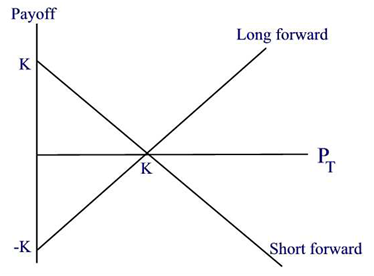# Payoff on Forward Contracts

Forward contracts are privately executed between two parties. The buyer of the underlying commodity or asset is referred to as the long side whereas the seller is the short side. The obligation to buy the asset at the agreed price on the specified future date is referred to as the long position. A long position profits when prices rise. The obligation to sell the asset at the agreed price on the specified future date is referred to as the short position. A short position profits when prices go down.

What is the payoff of a forward contract on the delivery date? Let T denote the expiration date, K denote the forward price, and PT denote the spot price (or market price) at the delivery date. Then

• For the long position: the payoff of a forward contract on the delivery date is PT_ K
• For the short position: the payoff of a forward contract on the delivery date is K _PT

Figure shows a payoff diagram on a contract forward. Note that both the long and short forward payoff positions break even when the spot price is equal to the forward price. Also note that a long forward’s maximum loss is the forward price whereas the maximum gain is unlimited.

For a short forward, the maximum gain is the forward price and the maximum loss is unlimited.Pay0ff diagrams show the pay0ff of a position at expiration. These payoffs do not include any costs or gains earned when purchasing the assets today. Payoff diagrams are widely used because they summarize the risk of the position at a glance. We have pointed out earlier that the long makes money when the price rises and the short makes money when the price falls.

Example

An investor sells 20 million yen forward at a forward price of \$0.0090 per yen. At expiration, the spot price is \$0.0083 per yen.

1. What is the long position payoff?
2. What is the short position payoff?

Solution.

(a) At the expiration date, the long position’s payoff is (0.0083 0.009) * 20.10^6 = -\$14; 000; a loss of \$14,000

(b) The short position’s payoff is \$14000 that is a profit of \$14,000

To check the introduction of Forward and future Contract You can read the following Article